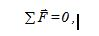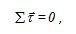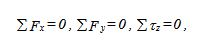# Equilibrium of a rigid

A static equilibrium condition is first studied experimentally and then theoretically. A meter stick, with small holes drilled at specific locations, is the rigid body and five forces are applied in order to produce a static condition that satisfies given constraints. Three of these forces have their magnitudes, directions, and points of application specified. The point of application the fourth force is variable and both the magnitude and direction of the fifth force are variable. These variable quantities are to be determined both experimentally and theoretically, and the results compared

The conditions for a rigid body to be in equilibrium are that the sum of the external forces acting on a rigid body must be equal to zero, known as the First Condition of Equilibrium; and that the sum of the external torques acting on a rigidbody must be equal to zeroknown as the Second Condition of Equilibrium. When the forces are confined to the x-y plane, (1) and (2) become